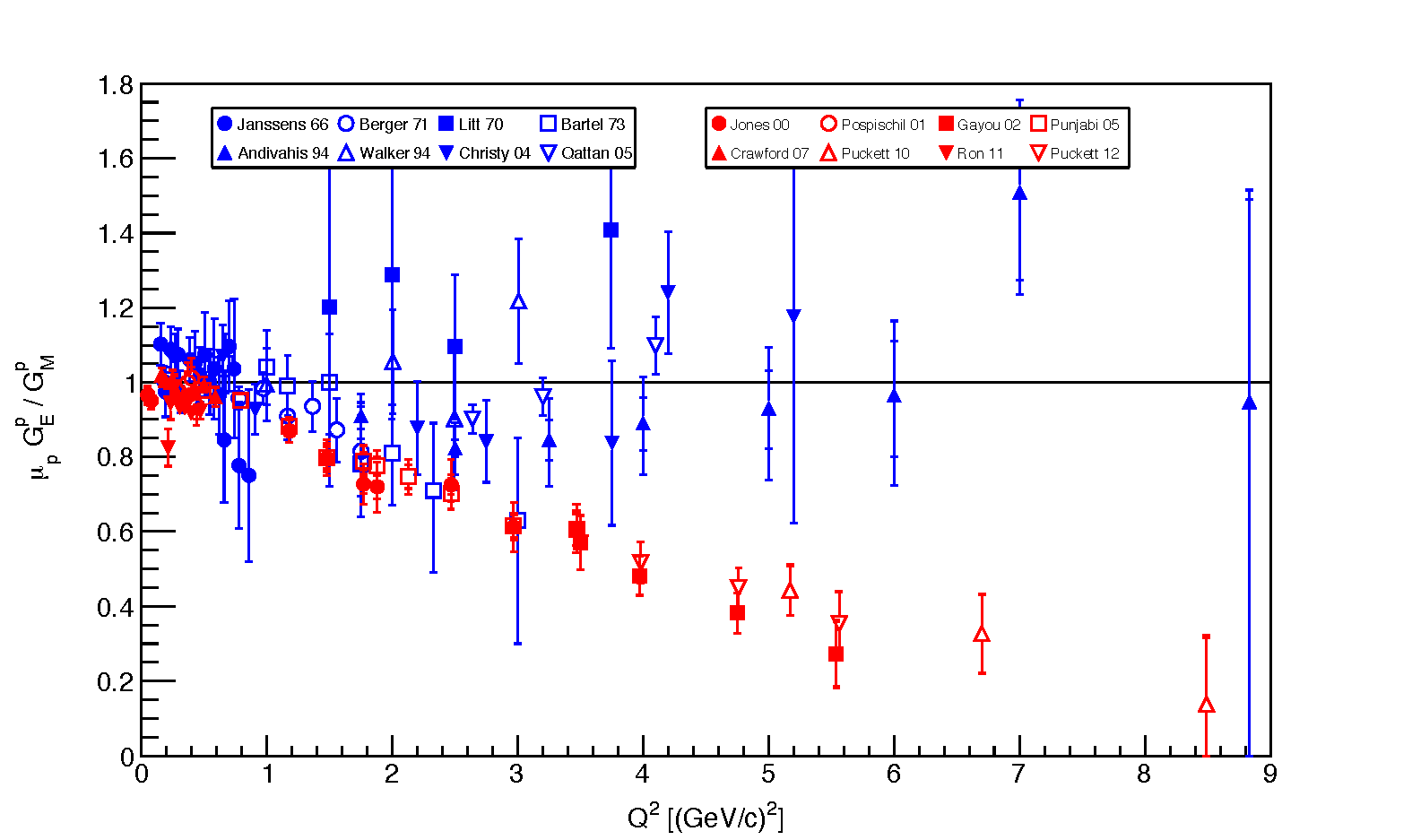## The OLYMPUS Experiment

### Motivation

The OLYMPUS experiment was proposed, designed, and carried-out to investigate the startling discrepancy in the proton form factor ratio observed at JLab in 2000 and in subsequent experiments.

The discrepancy is illustrated in the following figure showing the ratio of the proton's electric form factor, $GE$, to the proton's magnetic form factor, $GM$, versus the square of the momentum transfer, $Q2$ .The results shown in blue used the traditional Rosenbluth measurement technique while the results in red used polarized techniques.

The Rosenbluth results have large uncertainties inherent in the technique but largely cluster around unity with increasing momentum transfer. Historically, this led to the common interpretation that the electric and magnetic charge distributions inside the proton were similar and that both could be described by the same dipole form factor, which, in turn, could be explained as arising from an exponential distribution.

In contrast, the results obtained using the polarization techniques show a striking decrease with increasing $Q2$. By the nature of the technique the polarization measurements are more precise with smaller uncertainties.

How to explain this discrepancy?

A possible explanation is that multi-photon processes are important at higher momentum transfers. The unpolarized data sums over all processes and thus does not distinguish between single and multi-photon processes. But techniques using polarization are sensitive primarily to single photon exchange as the polarization information is, at some level, "washed-out" in multi-photon processes.

If multi-photon processes do play a significant role in elastic eletron scattering from nucleons then the unpolarized data must be re-evaluated and better electric and magnetic form factors extracted so that a correct model of the proton can be found for comparison with theoretical calculations.